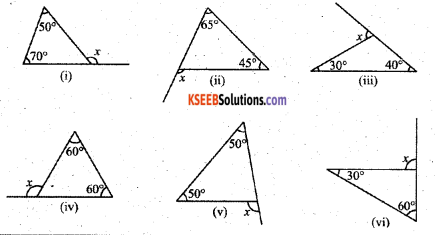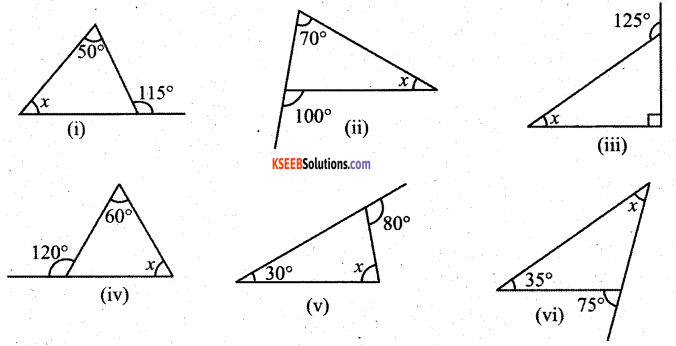# KSEEB Solutions for Class 7 Maths Chapter 6 The Triangles and Its Properties Ex 6.2

Students can Download Chapter 6 The Triangles and Its Properties Ex 6.2, Question and Answers, Notes Pdf, KSEEB Solutions for Class 7 Maths, Karnataka State Board Solutions help you to revise complete Syllabus and score more marks in your examinations.

## Karnataka State Syllabus Class 7 Maths Chapter 6 The Triangles and Its Properties Ex 6.2

Question 1.
Find the value of the unknown exterior angle x in the following diagrams :i) ∠x = 50° + 70° = 120°
(∵ The exterior angle of the triangle is equal to the sum of its two interior opposite angles.)

ii) ∠x = 45° + 65° = 110°
(∵ The exterior angle of the triangle is equal to the sum of its two interior opposite angles.)

iii) ∠x = 30° + 40° = 70°
(∵ The exterior angle of the triangle is equal to the sum of its two interior opposite angles.)

iv) ∠x = 60° + 60° = 120°
(∵ The exterior angle of the triangle is equal to the sum of its two interior opposite angles.)

v) ∠x = 50° + 50° = 100°
(∵ The exterior angle of a triangle is equal to the sum of its two interior opposite angles.)Question 2.
Find the value of the unknown interior angle x in the following figures:Solution:
i) ∠x + 50° = 115°
∵ The exterior angle of a triangle is equal to the sum of its two interior opposite angles.
∵ ∠x = 115° – 50° = 65°

ii) ∠x + 70° = 100°
Solution:
(∵ The exterior angle of a triangle is equal to the sum of its two interior opposite angles)
∴ ∠x = 100° – 70°= 30°iii) ∠x + 90° = 125°
∵ The exterior angle of a triangle is equal to the sum of its two interior opposite angles.
∴ ∠x = 125° – 90° = 35°

iv) ∠x + 60° = 120°
∵ The exterior angle of a triangle is equal to the sum of its two interior opposite angles.
∠x = 120° – 60° = 6o°

v) ∠x + 30° = 80°
∵ The exterior angle of a triangle is equal to the sum of its two interior opposite angles.
∵ ∠x = 80° – 30° = 50°

vi) ∠x + 35° = 75°
∵ The exterior angle of a triangle is equal to the sum of its two interior opposite angles.
∴ ∠x = 75° – 35° = 40°